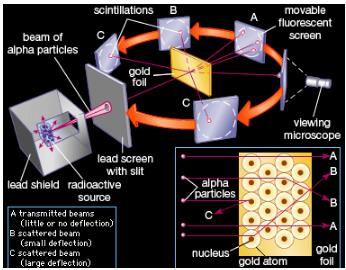Courses

# Test: Scattering of Alpha Particles

## 10 Questions MCQ Test Physics For JEE | Test: Scattering of Alpha Particles

Description
This mock test of Test: Scattering of Alpha Particles for JEE helps you for every JEE entrance exam. This contains 10 Multiple Choice Questions for JEE Test: Scattering of Alpha Particles (mcq) to study with solutions a complete question bank. The solved questions answers in this Test: Scattering of Alpha Particles quiz give you a good mix of easy questions and tough questions. JEE students definitely take this Test: Scattering of Alpha Particles exercise for a better result in the exam. You can find other Test: Scattering of Alpha Particles extra questions, long questions & short questions for JEE on EduRev as well by searching above.
QUESTION: 1

### Which of these is true?

Solution:

Rutherford declared that the "nucleus" (as he now called it) was indeed positively charged, based on the result of experiments exploring the scattering of alpha particles in various gases.

QUESTION: 2

### Which of the following is/are deduced from the Rutherford’s scattering experiment? (1) There are neutrons inside the nucleus. (2) The sign of the charge of the nuclei is the same as the sign of alpha particles. (3) Electrons are embedded in the nucleus.​

Solution:

When he sent alpha particles during his gold foil experiment he observed that most of the rays moved away from the nucleus.As we know that like charges always repel ,he concluded that the charge the nuclei is same as the charge of alpha particles

QUESTION: 3

### In scattering, the impact parameter b is defined as the:

Solution:

The impact parameter is defined as the perpendicular distance between the path of a projectile and the center of a potential field created by an object that the projectile is approaching. It is often referred to in nuclear physics (see Rutherford scattering) and in classical mechanics.

QUESTION: 4

The alpha particle scattering experiment was carried out by:

Solution:

Rutherford Alpha Particle Scattering Experiment.Rutherford directed beams of alpha particles (which are the nuclei of helium atoms and hence positively charged) at thin gold foil to test this model and noted how the alpha particles scattered from the foil.

QUESTION: 5

We know that the Rutherford model of the atom is superior to the Thompson model because when alpha particles are scattered from atoms:

Solution:

In ruther ford experiment he suggest that all the positive charge and mass are concentrated at the centre when he bombarded the alpha partical which is dipositive in nature and when it is more close to centre it get deflect to a large angle and with increase of closenes to centre its deflection angle increase and some alpha partical deflect to 180 degree so it prove that all the positive charge and mass are concentrated at the centre where as acccording to thomson atom is hard solid sphere in which its total +ve charge and mass uniformalyy distributed on the surface and electrone reside as seed in watermelon ( plum pudding model)

QUESTION: 6

In Rutherford’s experiment, a thin gold foil was bombarded with alpha particles. According to Thomson’s “plum-pudding” model of the atom, what should have happened?

Solution:

In Rutherford's experiment, a thin gold foil was bombarded with alpha particles. According to Thomson's "plum-pudding" where atoms are to be made up of electrons embedded in positive charge cloud, Alpha particles should have passed through the foil with little or no deflection because the diffused, massless positive charge cloud will not pose any obstacle to the fast moving heavy alpha particle beam.

QUESTION: 7

The distance of closest approach when a 15.0 MeV proton approaches gold nucleus (Z = 79) is​

Solution:

Explanation : E = 15.0MeV

= 15 * 106 eV

= 15 * 106 * 1.6 * 10-19 J

= 15 * 1.6 * 10-13 J

E = (1/4πεo)*(ze2/r02)

r0 = (1/4πεo)*(ze2/E)

r0 = (9*109*79*(1.6*10-19)2)/(15*1.6*10-13)

= 75.84 * 10-16 m

= 7.58 fm

QUESTION: 8

The targets used in the alpha particle atomic experiments in the early 1900’s was:

Solution:Physicist Ernest Rutherford established the nuclear theory of the atom with his gold-foil experiment. When he shot a beam of alpha particles at a sheet of gold foil, a few of the particles were deflected. He concluded that a tiny, dense nucleus was causing the deflections.

QUESTION: 9

Rutherford’s experiments on scattering of alpha particles proved that:

Solution:

Most of the α-particle passed through the foil straight without suffering any change in their direction. This shows that most of the space inside the atom is empty or hollow.
A small fraction of α-particles was deflected through small angles and a few through larger angles. For this to happen α- particles (positively charged) must approach a heavy positively charged core inside the atom (like charges repel each other). This heavy positively charged core inside the atom was named as the nucleus.

QUESTION: 10

Number of alpha particles N scattered at an angle θ during Rutherford’s alpha scattering experiment is :

Solution:

Solution :- For a single scatterer, such as a single gold nucleus within a thin gold foil layer, the differential scattering cross section is defined as follows :

dσ(θ, φ)/dΩ = flux scattered into element dΩ at angles (θ, φ)/incident flux per unit area

dσ/dΩ = (Iθ × A)/ (dΩ × I0 × NAvo × ρ × x(foil))........... (1)

where NAvo is Avogadro’s number, xfoil is the thickness of the target foil, A is the atomic mass of the material in the target foil, dΩ is the solid-angle of the detector, I0 is the unattenuated intensity of the alpha particle beam.

dσ/dΩ = [(ZZ0 e^2/4E )^2]/(1/sin^4(θ/2 ))

dσ/dΩ is directly proportional to 1/sin^4(θ/2 )